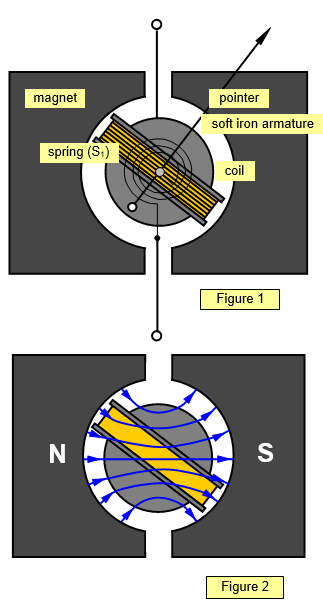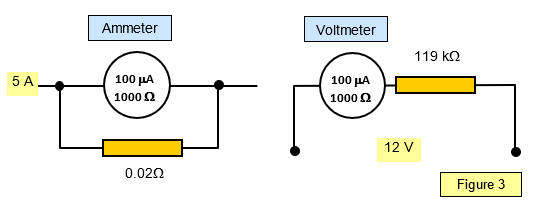# The moving coil meterA very common use of the forces on a coil in a magnetic field is that of the moving coil meter, shown in Figure 1.

The coil is suspended between the poles of a magnet on jewelled bearings and is held in place by two finely coiled springs (S1 and S2) through which the current to be measured passes in and out of the coil.

The pole pieces are shaped so that the magnetic field is radial thus giving the maximum and constant torque on the coil whatever its position (Figure 2). There is a soft iron armature in the centre of the coil, and this further concentrates the magnetic field through it due to the high value of the relative permeability of the material of the core.

The coil is supported on a light metal frame and induced currents in this frame give electromagnetic damping of the movement.
When it is in equilibrium with a current passing through it, the torque on the coil produced by the magnetic field is balanced by an opposing torque due to the rigidity of the springs. Clearly the more delicate the springs the bigger the deflection for a given current.

Some meters are of the suspended coil type; the coil is mounted on a fine phosphor bronze wire and the deflection measured with a lamp and scale. A small mirror mounted above the coil reflects a beam of light on to a scale, the angle of twist of the light beam being double the angle of rotation of the coil. Such instruments have a framework of brass or aluminium.

Meters are often made with a protective series resistor since too large a current will burn out the springs or the coil. When the correct range for the meter has been found this protective resistor may be shorted out.

The moving coil analogue meter is used less and less these days having been replaced in many applications by the digital meter.

## Sensitivity of a meter

The amount of deflection of the pointer in a meter for a certain current depends on the design of the meter. The amount of angular twist for a unit (1A) current is called the current sensitivity of the meter.

Consider a coil of N turns and cross-sectional area A, carrying a current I in a field of flux density B. The torque C due to the magnetic field is given by

Torque (C) on the coil = BANI

for a radial field, and the opposing torque due to the twist of the suspension is kq where k is the torsion constant for the wire and q is the angle of twist.
In equilibrium kq = BANI

The angular twist per unit current (q/I) is called the current sensitivity of the meter and is defined by the formula:

Current sensitivity (θ/I) = BAN/k

To increase this (that is, to make the meter more sensitive) we require:
(a) large magnetic flux density (B), that is, the gap between the poles as small as possible,
(b)a coil with a large area (A),
(c) a large number of turns (N), and
(d) a small value of k that is, a very thin wire or one with a very low rigidity.
Unfortunately (b) and (c) tend to make the coil both bigger and heavier and so cause problems with (a) and (d). A compromise has to be reached.
The radial field is important since for this arrangement B sinθ is constant and therefore the angle of twist <θ is directly proportional to the current I for all positions of the coil.

Example problem
The coil of a lamp and scale galvanometer has an area of 4 cm2 and 200 turns.
A torque of 2x10-7 Nm causes it to twist through 180o against the torsion of the suspension. If the field acting on the coil is 0.2 T, find the current that will cause the spot of light on a scale 1 m away to be deflected through 1 mm.

Original torque = 2x10-7 = kqo.

Therefore k = 2x10-7/qo.
New angle (q) is given by: q/I = [0.2x4x10-4 x 200 x p]/2x10-7
Angle of deflection = 10-3/1= 10-3 radians
Therefore current I = 2 x 10-7 x 10-3/0.2 x 4x10-4 x 200 x p = 2x10-10/5.02x10-2 = 4x10-9 A = 4 x 10-3 mA

## Conversion to ammeter or voltmeter

A typical moving coil meter such as those used in schools may have a full scale deflection (fsd) of 100 mA and a resistance of 1000 W. This means that the pointer will deflect right across the scale when a current of 100 mA is passed through the meter.
When this occurs the p.d. between the terminals of the meter will be 100 mA x 1000 W = 100 mV. Clearly this is quite inadequate when measurements of currents of say 5 A or voltages of 12 V are required. External resistors may be used to extend the range of the meter and these are known as shunts and series resistors.
(see Figure 2)So that as little current as possible is drawn from a circuit under test a good voltmeter should have a resistance of at least 1000W per volt - for example a meter designed to read. voltages up to 10 V should have a resistance of 10 kW. This also requires a galvanometer with a high current sensitivity

The moving coil galvanometer can also be used as an ohmmeter and a wattmeter.

## The digital meterThere has been a considerable increase in the use of direct-reading digital meters in the last few years. These rely on a totally different principle, that of the integrated circuit, for their operation and have no moving parts. The digital voltmeter has a very high resistance (of the order of 10 MW on d.c.) but it does need a small internal battery to power the instrument.

The input voltage to be measured is compared with a steadily rising voltage produced by a ramp generator (an application of the op amp). The time taken for the rising voltage to reach that of the input voltage is measured and this time is directly proportional to the voltage. The output reading is scaled to give a direct reading in volts.

## Measurement of alternating current

It should be clear that none of the preceding moving coil instruments are suitable for the measurement of alternating current or voltage.
The coil would tend to oscillate between a positive and a negative reading. In Britain, however, the frequency of the mains is 50 Hz and the inertia of a coil will prevent it from moving far before the current reverses, so it simply vibrates slightly.

The following types of meter can be used to measure alternating currents:
moving iron instruments - using the repulsion between two metal rods in the field
hot wire instruments - using the expansion of a wire rectifier instruments
dynamometer instruments electrostatic voltmeter
diode valve voltmeter
cathode ray oscilloscope
digital meter

A VERSION IN WORD IS AVAILABLE ON THE SCHOOLPHYSICS USB